# Congruence condition on prime divisor of cyclotomic polynomial evaluated at an integer

Jump to: navigation, search

## Statement

Supposeis an integer,is a natural number, anddenotes the cyclotomic polynomial for the primitiveroots of unity. Supposeis a prime divisor of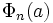. Then, eitheris congruent tomodulo, or we can write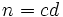, wheredivides, andis congruent tomodulo.

In particular, at least one of these two conditions must hold:

•divides.
•is congruent tomodulo.

## Proof

Given: An integer, a natural number. A prime divisorof.

To prove:dividesor <we can writesuch thatdividesandis congruent tomodulo.

Proof: Since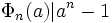,andare relatively prime and the order ofmodulodivides.

Letbe the order ofmodulo. Then,divides. Let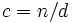. Write: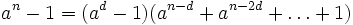.

We now consider two cases:

• Case 1: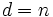. In this case, the order ofmodequals. But by Fermat's little theorem, the order ofmoddivides. Thus,is congruent tomodulo.
• Case 2: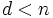. In this case, no primitiveroot of unity is aroot of unity. Now,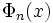divides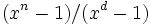(one way of seeing this is thatis the product of linear factors for primitiveroots of unity, whileis the product of linear factors forroots that aren'troots. In particular,divides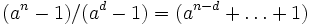. Each of the monomials in the right side is a power of, hence ismod, and there areterms. Thus,divides. Also, the order ofmoddivides, soismodulo. This completes the proof.